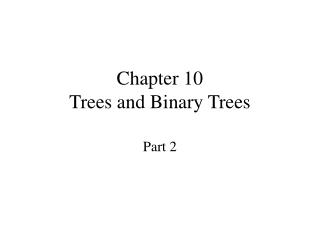DownloadDownload PresentationChapter 10 Trees and Binary Trees

Chapter 10 Trees and Binary Trees

Télécharger la présentationChapter 10 Trees and Binary Trees

- - - - - - - - - - - - - - - - - - - - - - - - - - - E N D - - - - - - - - - - - - - - - - - - - - - - - - - - -
Presentation Transcript

1. ?Traversal level by level

2. Definitions

3. Rooted trees with four vertices(Root is at the top of tree.)

4. Ordered trees with four vertices

5. Implementations of Ordered Trees • Multiple links • first child and next sibling links • Correspondence with binary trees

6. Conversion (from forest/Orchard to binary tree Corresponded binary tree Corresponded binary tree respectively

7. Huffman Tree(哈夫曼树) Path Length (路径长度 ) Path Length of the binary tree Total length of all from leaves to root

8. Weighted Path Length(WPL,带权路径长度 ) 树的带权路径长度是树的各叶结点所带的权值与该结点到根的路径长度的乘积的和。

9. How about the WPLof the following binary trees

10. Huffman tree A (extended) binary tree with minimal WPL。

11. Processes of huffman tree

12. Huffman coding Compression suppose we have a message： CAST CAST SAT AT A TASA alphabet ={ C, A, S, T }，frequency of them (次数) are W＝{ 2, 7, 4, 5 }。 first caseequal length coding A : 00 T : 10 C : 01 S : 11 Total coding length of the message is ( 2+7+4+5 ) * 2 = 36.

13. Huffman tree ?? A : 0 T : 10 C : 110 S : 111 Total length of huffman coding： 7*1+5*2+( 2+4 )*3 = 35 Which is shorter than that of equal length coding。 霍夫曼编码是一种无前缀编码。解码时不会混淆。

14. Binary Search Trees • Can we find an implementation for ordered lists in which we can search quickly (as with binary search on a contiguous list) and in which we can make insertions and deletions quickly (as with a linked list)?

15. DEFINITION • A binary search tree is a binary tree that is either empty or in which the data entry of every node has a key and satisfies the conditions: 1. The key of the left child of a node (if it exists) is less than the key of its parent node. 2. The key of the right child of a node (if it exists) is greater than the key of its parent node. 3. The left and right subtrees of the root are again binary search trees. We always require: No two entries in a binary search tree may have equal keys.

16. different views • We can regard binary search trees as a new ADT. • We may regard binary search trees as a specialization of binary trees. • We may study binary search trees as a new implementation of the ADT ordered list.

17. The Binary Search Tree Class

18. Recursive auxiliary function: template <class Record> Binary node<Record> *Search tree<Record> :: search for node( Binary node<Record>* sub root, const Record &target) const { if (sub root == NULL || sub root->data == target) return sub root; else if (sub root->data < target) return search for node(sub root->right, target); else return search for node(sub root->left, target); }

19. Nonrecursive version: template <class Record> Binary node<Record> *Search tree<Record> :: search for node( Binary node<Record> *sub root, const Record &target) const { while (sub root != NULL && sub root->data != target) if (sub root->data < target) sub root = sub root->right; else sub root = sub root->left; return sub root; }

20. Public method for tree search: template <class Record> Error code Search tree<Record> :: tree search(Record &target) const { Error code result = success; Binary node<Record> *found = search for node(root, target); if (found == NULL) result = not present; else target = found->data; return result; }

21. Binary Search Trees with the Same Keys

22. search

23. Creating a BST by insertion

24. insertion

25. Method for Insertion

26. Method for Insertion

27. Analysis of insertion

28. Treesort • When a binary search tree is traversed in inorder, the keys will come out in sorted order. • This is the basis for a sorting method, called treesort: Take the entries to be sorted, use the method insert to build them into a binary search tree, and then use inorder traversal to put them out in order.

29. Treesort

30. Comparison First advantage of treesort over quicksort: The nodes need not all be available at the start of the process, but are built into the tree one by one as they become available. Second advantage: The search tree remains available for later insertions and removals. Drawback: If the keys are already sorted, then treesort will be a disasteróthe search tree it builds will reduce to a chain. Treesort should never be used if the keys are already sorted, or are nearly so.

31. Removal from a Binary Search Tree

32. Removal from a Binary Search Tree (continue)

33. Height Balance: AVL Trees Definition: An AVL treeis a binary search tree in which the heights of the left and right subtrees of the root differ by at most 1 and in which the left and right subtrees are again AVL trees. With each node of an AVL tree is associated a balance factor that is left higher, equal, or right higher according, respectively, as the left subtree has height greater than, equal to, or less than that of the right subtree.

34. Example AVL trees

35. Example AVL trees

36. Example non-AVL trees

37. Example non-AVL trees

38. Insertions into an AVL tree

39. Insertions into an AVL tree

40. Rotations of an AVL Tree

41. Double Rotation

42. Deletion with no rotations

43. Deletion with single left rotations

44. Deletion with double rotation

45. Worst-Case AVL Trees